# Difference between revisions of "Path-connected space"

A topological space in which any two points can be joined by a continuous image of a simple arc; that is, a spacefor any two pointsand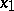of which there is a continuous mappingof the unit intervalsuch that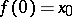and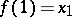. A path-connected Hausdorff space is a Hausdorff space in which any two points can be joined by a simple arc, or (what amounts to the same thing) a Hausdorff space into which any mapping of a zero-dimensional sphere is homotopic to a constant mapping. Every path-connected space is connected (cf. Connected space). A continuous image of a path-connected space is path-connected.
Path-connected spaces play an important role in homotopic topology. If a spaceis path-connected and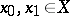, then the homotopy groups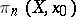andare isomorphic, and this isomorphism is uniquely determined up to the action of the group. If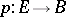is a fibration with path-connected base, then any two fibres have the same homotopy type. Ifis a weak fibration (a Serre fibration) over a path-connected base, then any two fibres have the same weak homotopy type.
The multi-dimensional generalization of path connectedness is-connectedness (connectedness in dimension). A spaceis said to be connected in dimensionif any mapping of an-dimensional sphereinto, where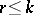, is homotopic to a constant mapping.